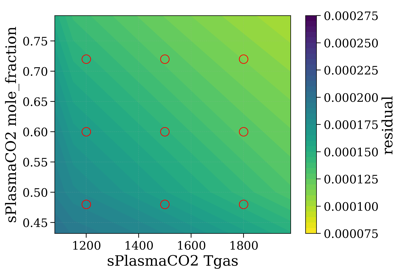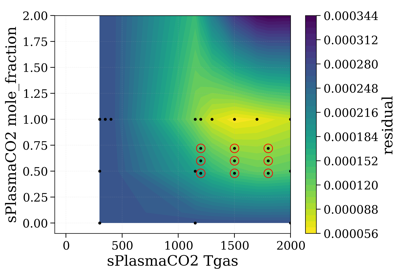# fitroom package¶

## Module contents¶

class CaseSelector(dbInteractx=None, dbInteracty=None, xparam='', yparam='', slbInteractx=None, slbInteracty=None, nfig=None, xmin=0, xmax=0, ymin=0, ymax=0, plot_data_color='k')[source]

Bases: `object`

Main tool to choose which cases to plot

Examplesconnect(fitroom)[source]

Triggered on connection to FitRoom

line_select_callback(eclick, erelease)[source]

eclick and erelease are the press and release events

precompute_residual(Slablist, xspace='database', yspace='database', contour='contourf', normalize=False, normalize_how='max', vmin=None, vmax=None)[source]

Plot residual for all points in database.

Parameters
• Slablist (configuration)

• xspace (array, or ‘database’) – values of points to precompute. If ‘database’, residual is calculated for all points in database. Default ‘database’.

• yspace (array, or ‘database’) – values of points to precompute. If ‘database’, residual is calculated for all points in database. Default ‘database’.

Other Parameters

vmin, vmax (float) – used for colorbar

Examples

When `yparam` is mole_fraction and we want to calculate for many mole fraction conditions from 0 to 1.

```selectTool.precompute_residual(Slablist, normalize=normalize,
yspace=np.linspace(0.1, 1, 10))
```
toggle_selector(event)[source]
update_action(xmin, xmax, ymin, ymax)[source]
update_markers(fconfig, i=1, j=1)[source]
Parameters

i,j (int) – marker position (different from 1,1 in 3x3 grid )

update_target_config(xcen, ycen)[source]
class DynVar(slab, param, func=<function DynVar.<lambda>>)[source]

Bases: `object`

To allow dynamic (runtime) filling of conditions in SlabList

Parameters
• slab (str) – slab config name in Slablist. Note that `'self'` in DynVar can be used to refer to the DynVar’s own slab. Ex:

```slabPlasma={
'Trot':2000,
'Tvib':DynVar('self', 'Trot'),
}
```
• param (str) – param name in slab config dict

• func (function) – function to apply. Default identity

Examples

``` slbPostCO2 = {
'db':db0,
'Tgas':500,
'db':dbp,
'Tgas':1100,
'path_length':0.7,
'mole_fraction':0.35,
}

slbPostCO = {
'db':dbco,
'Tgas':slbPostCO2['Tgas'],
'path_length':DynVar('sPostCO2', 'path_length', lambda x:x),
# evaluated at runtime using names in Slablist
'mole_fraction':0.01,
}

Slablist = {
'sPostCO2': slbPostCO2,
'sPostCO': slbPostCO,
}
```

You can also use a function, for instance to maintain the equilibrium concentration in a fit on temperature:

```from radis.tools.gascomp import get_eq_mole_fraction
get_co2_eq = lambda T: get_eq_mole_fraction('CO2:1', T, 1e5)
slbPlasmaCO2 = {
'db':dbp,
'Trot':1500,
'Tvib':DynVar('self', 'Trot'),
'path_length':0.025,
'mole_fraction':DynVar('self', 'Trot', get_co2_eq),
}
```

Here the CO2 Plasma slab is always evaluated with Tvib=Trot and x_co2 at chemical equilibrium with T=Trot.

eval(slabsconfig)[source]

Evaluate value at runtime based on other static values

class FitRoom(Slablist, slbInteractx, slbInteracty, xparam, yparam, perfmode=False)[source]

Bases: `object`

Parameters
• perfmode (boolean)

• if ``True`` we try to optimize calculation times (ex (minimized windows)

• are not recalculated)

Exampleseval_dynvar(config)[source]

Evaluate dynamic links for a given configuration. Changes updated config (inplace) Note that ‘self’ in DynVar can be used to refer to the current slab

get_config()[source]

Get values for Target configuration

update(xspace=None, yspace=None)[source]
update_plots()[source]
class Grid3x3(slbInteractx=None, slbInteracty=None, xparam='', yparam='', plotquantity='radiance', unit='mW/cm2/sr/nm', wunit='nm', normalizer=None, s_exp=None)[source]

Bases: `object`

Where the output of a `CaseSelector` is shown.

Examplescalc_case(i, j, **slabsconfig)[source]

notice j, i and not i, j i is y, j is x? or the other way round. It’s always complicated with indexes anyway… (y goes up but j goes down) you see what i mean it works, anyway

connect(fitroom)[source]

Triggered on connection to FitRoom

format_coord(x, y)[source]
plot_3times3(xspace=None, yspace=None)[source]
plot_all_slabs(s, slabs)[source]
plot_case(i, j, ax_out=None, plot_all_labels=False, **slabsconfig)[source]

notice j, i and not i, j i is y, j is x? or the other way round. It’s always complicated with indexes anyway… (y goes up but j goes down) you see what i mean it works, anyway

Other Parameters
• ax_out (ax) – if None, plot to the GridTool. Else, plot to this ax (used for export)

• plot_all_labels (bool) – force plot all labels

plot_for_export(style='origin', cases=[], ls='-', lw=1, xlim=None, ylim=None, labelvar='xy', color=None, labelunit='K', cutwings=0, kwargs_exp={})[source]

Sum all center column in one case.

Parameters
• cases (list) – list of [(row, column)] to plot. If `None` or [], use a vertical line, i.e.

```cases=[(1,1), (0,1), (2,1)]
```
• ls (str (‘-‘, ‘-.’, etc.), list, or dict) – if str, use the same. If list, rotate. If dict, use `cases` as keys.

the first one is plot in solid line, the others in alternate with ‘-.’, ‘:’, ‘-.’

Other Parameters
• labelvar (‘x’, ‘y’, ‘xy’) – which variable to add. default ‘xy’ Ex:

```Tvib=, Trot=
Tvib=
Trot=
```
• cutwings – see `plot_stack()`

• kwargs_exp (dict) – parameters forwarded to `plot_stack` to plot the experiment

plot_for_export_1times3(cases=[], ls='-', lw=1, xlim=None, ylim=None, labelvar='xy', color=None, labelunit='K', cutwings=0, kwargs_exp={})[source]
update_markers(fconfig, i, j)[source]
update_slit()[source]
class MultiSlabPlot(plotquantity='radiance', unit='mW/cm2/sr/nm', normalizer=None, s_exp=None, nfig=None, N_main_bands=5, keep_highlights=False, show_noslit_slabs=True, show_slabs_with_slit=True, wunit='nm')[source]

Bases: `object`

Plot the center case in `CaseSelector` by also showing emission and absorption separately, and all slabs

Parameters
• N_main_bands (int)

• show main emission bands in case an overpopulation tool is defined.

• N_main_bands is the number of bands to show. Default 5.

• keep_highlights (boolean)

• if ``True``, delete previous highlights when generating new case. Keeping

• them can help remember the last band position. Default ``False``.

• show_noslit_slabs (boolean)

• if ``True``, overlay slabs with non convoluted radiance / transmittance

• show_slabs_with_slit (boolean)

• if ``True``, slit is applied to all slabs before display (this does not

• change the way the radiative transfer equation is solved)

Other Parameters

wunit (‘nm’, ‘cm-1’)

Examplesconnect(fitroom)[source]

Triggered on connection to FitRoom

format_coord(x, y)[source]
plot_broken(style=['origin'], xlim=None, lw_multiplier=1, skip_exp_range=[], cutwings=0, verbose=True)[source]

Not used in Fitroom, but can be used by user to export / save figure with broken axes

Parameters

xlim (tuple, or list of tuple) – if None, all range is plot. If tuple, only this range is plot. If list of tuple, broken axes are used. Only works for `fig0` though. Not Implemented for `fig1`.

Other Parameters
• lw_multiplier (float) – multiply line widths

• skip_exp_range ([(wmin, wmax), (wmin2, wmax2), etc.]) – dont plot these ranges for experimental spectrum. Default []

• cutwings – see `plot_stack()`

Examples

```fig0 = slabsTool.plot_broken()
fig0.savefig('...')
```
plot_for_export(style=['origin'], lw_multiplier=1, skip_exp_range=[], cutwings=0, figsize=[(20, 4), (20, 6.5)])[source]

Not used in Fitroom, but can be used by user to export / save figures

Other Parameters
• lw_multiplier (float) – multiply line widths

• skip_exp_range ([(wmin, wmax), (wmin2, wmax2), etc.]) – dont plot these ranges for experimental spectrum. Default []

• cutwings – see `plot_stack()`

Examples

```fig0, fig1 = slabsTool.plot_for_export()
fig0.savefig('...')
```
update()[source]

Get, calculate and plot the current config

update_markers(fconfig)[source]
update_slit()[source]
class Overpopulator(slab, levels='all', nfig=None)[source]

Bases: `object`

Parameters

slab – slab to connect to. Must be a slab calculated with from_band source mode

Examples

action()[source]
connect(fitroom)[source]

Triggered on connection to FitRoom

get()[source]
update_overpopulation()[source]
class SlabsConfigSolver(config, source=None, s_exp=None, plotquantity='radiance', unit='mW/cm2/sr/nm', slit=None, slit_options='default', crop=None, retrieve_mode='safe', verbose=True, retrieve_error='ignore')[source]

Bases: `object`

Machinery related to solving a specific Slabs configuration: parse the database, get the correct slab input, then calls the appropriate functions in neq.spec engine

Parameters
• config (dict) – list of Slabs that represent the Spatial model (to solve RTE)

• source (‘database’, ‘calculate’, ‘from_bands’) – Whether to calculate spectra from scratch, retrieve them from a database, or combine vibrational bands Mode can be overriden by a ‘source’ parameter in every slab

• s_exp (`Spectrum`) – experimental spectrum

Other Parameters
• get_closest (bool) – when retrieving spectra from database, get cloest if set to True. Else get unique.

• slit_options

if `'default'`, use:

```{'norm_by':'area', 'shape':'triangular', 'unit':'nm', 'verbose':False}
```

and adapt `'shape'` to `'trapezoidal'` if a tuple was given for slit

• crop (tuple, or None) – if not `None`, restrain to the given fitted interval.

• retrieve_error (‘ignore’, ‘raise’) – if Spectrum cannot be calculated or retrieved from Database, then returns `None` as a Spectrum object. The rest of the code should deal with it. Else, raises an error immediatly.

• retrieve_mode (‘safe’, ‘strict’, ‘closest’) –

how to retrieve spectra when reading from database:

• if ‘strict’, only retrieve the spectra that exactly match

the given conditions (allow scaling path_length or mole_fraction, still)

• if ‘safe’, requires an exact match for all conditions (as in

‘strict’), except for the 2 user defined variable conditions `xparam` and `yparam`

• if ‘closest’, retrieves the closest spectrum in the database

Warning

‘closest’ can induce user errors!!!. Ex: a Trot=1500 K spectrum can be used instead of a Trot=1550 K spectrum if the latter is not available, without user necessarily noticing. If you have any doubt, print the conditions of the spectra used in the tools. Ex:

```for s in gridTool.spectra:
print(s)
```

ExamplesParameters

slabsconfig – list of dictionaries. Each dictionary as a database key `db` and as many conditions to filter the database

connect(fitroom)[source]

Triggered on connection to FitRoom

Returns difference between experimental and simulated spectra By default, uses `get_residual()` function You can change the residual by overriding this function.

Examples

Replace get_residual with new_residual:

```solver.get_residual = lambda s: new_residual(solver.s_exp, s, 'radiance')
```

Note that default solver would be written:

```from radis import get_residual
solver.get_residual = lambda s: get_residual(solver.s_exp, s,
solver.plotquantity,
ignore_nan=True)
```
Parameters
• s (Spectrum object) – simulated spectrum to compare with (stored) experimental spectrum

• normalize (bool) – not implemented yet # TODO

Notes

Implementation:

interpolate experimental is harder (because of noise, and overlapping) we interpolate each new spectrum on the experiment

retrieve_error[source]

‘ignore’, ‘raise’

Type

str

retrieve_mode[source]

str ‘safe’, ‘strict’, ‘closest’

class SlitTool(plot_unit='nm', overlay=None, overlay_options=None)[source]

Bases: `object`

Tool to manipulate slit function

Parameters
• plot_unit (‘nm’, ‘cm-1’)

• overlay (str, or int, or tuple) – plot a slit in background (to compare generated slit with experimental slit for instance)

Examplesconnect(fitroom)[source]

Triggered on connection to FitRoom

plot_slit(w, I=None, waveunit='', plot_unit='same', wover=None, Iover=None)[source]

Variant of the plot_slit function defined in slit.py that can set_data when figure already exists

Plot slit, calculate and display FWHM, and calculate effective FWHM. FWHM is calculated from the limits of the range above the half width, while FWHM is the equivalent width of a triangular slit with the same area

Parameters
• w, I (arrays or (str, None)) – if str, open file directly

• waveunit (‘nm’, ‘cm-1’ or ‘’)

• plot_unit (‘nm, ‘cm-1’ or ‘same’) – change plot unit (and FWHM units)

• warnings (boolean) – if `True`, test if slit is correctly centered and output a warning if it is not. Default `True`

slit_function()[source]
slit_options()[source]
update_figure()[source]
update_slider(val)[source]

update slit function in Solver and replot